Language
(Click to change)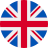*Auto Translated
Currency
(Click to change)
\$
Share It: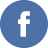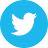26 Sep 2023 22:36:12 UTC

# LOTTERY VALIDATION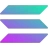Solana (SOL)
Week: Sep. 25, 2022
PREDICTED WINNING NUMBER
The predicted winning number changes each time a new player added and verified.
Not available. No players.
BASIC SEED
Random number of up to 128 digits, generated at the beginning of each week for each lottery.
40821827646410526278900637367195448229438885269057383220038263680445637842225666703393784064753569546722713053395593055605371798
TRANSACTION ID
Transaction ID of the last verified player.
Not available. No players.
TICKETS
Number of lottery tickets sold.
0
PLAYERS
Number of verified players.
0

## How is the Winning Number Generated?

Lottocryptos uses a "Provably Fair" system for its lotteries to achieve transparency in the generation of winning numbers.

The seed of the winning random number generation is composed of four parts:

• A - Random number up to 128 digits. It is generated weekly when the lottery begins and is different for each cryptocurrency.
• B - The transaction ID of the last player. The transaction ID of the last verified player of the lottery.
• C - The total number of lottery tickets sold in the lottery.
• D - The number of verified players in the lottery.
• To obtain the seed for the random generation of the winning number, the following process is followed.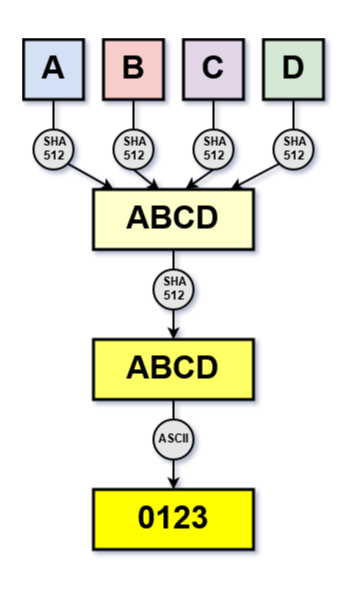Each part is encrypted with SHA512 and concatenated. Then it is encrypted again with SHA512 and its characters are converted to their corresponding ASCII value. The seed used for the generation of the random number is this value.

In order to be able to make all the checks considered appropriate, we provide users with the source code used to obtain the random number and the tools to perform a randomness test.

SOURCE CODE
File: classes/class.Random.php
PHP Version: 8.0.18
Last Update: Sep. 17, 2022 10:57:44 UTC
Size: 3231 Bytes
``` <?php function Random_number(\$length){     \$result = '';     //Generate random number.         for(\$i=0;\$i<\$length;\$i++){         \$result = \$result.random_int(0,9);     }          //Remove leading zeros.         \$result = ltrim(\$result,'0');     if(\$result == ''){         \$result = 0;     } return \$result;     } function Random_string_to_int(\$string){     \$result = '';     //Count characters.     \$length = strlen(\$string);          //Ord ->Convert a character to its ASCII decimal value.     for(\$i=0;\$i<\$length;\$i++){         \$result = \$result.ord(\$string[\$i]);      } return \$result;     } function Random_int_to_string(\$number){     //Convert integer to string.         \$result = number_format(\$number,0,'',''); return \$result;     } function Random_hash(\$data,\$algo){     //Hash string with an algo.         \$result = hash(\$algo,\$data); return \$result;     } function Random_basic_seed(){     \$result = Random_number(128); return \$result; } function Random_gmp_winner_number(\$basic_seed,\$player_seed,\$n_tickets,\$n_players){     \$result = '';     //Convert integer to string.     \$n_tickets = Random_int_to_string(\$n_tickets);          //Hash all the parts     \$basic_seed = Random_hash(\$basic_seed,'sha512');     \$player_seed = Random_hash(\$player_seed,'sha512');     \$n_tickets_seed = Random_hash(\$n_tickets,'sha512');     \$n_players_seed = Random_hash(\$n_players,'sha512');                  //Join all parts and hash     \$seed = Random_hash(\$basic_seed.\$player_seed.\$n_tickets_seed.\$n_players_seed,'sha512');          //Convert letters to numbers.     \$seed = Random_string_to_int(\$seed);          //Insert seed into number generator. Only integers.     gmp_random_seed(\$seed);          //Generate random number.     \$value = gmp_random_range(1,\$n_tickets);          //Convert GMP integer to string.         \$result = gmp_strval(\$value); return \$result; } /* //WINNER NUMBER \$basic_seed = Random_basic_seed(); \$player_seed = 'f4184fc596403b9d638783cf57adfe4c75c605f6356fbc91338530e9831e9e16';//Transaction ID \$n_players = 1; \$n_tickets = 100000000; echo Random_gmp_winner_number(\$basic_seed,\$player_seed,\$n_tickets,\$n_players); //RANDOMNESS TEST (Can be CPU intensive with more cycles / rounds) \$cycles = 1; \$runs = 1000; \$tickets = 5; \$player_seed = 'f4184fc596403b9d638783cf57adfe4c75c605f6356fbc91338530e9831e9e16';//Transaction ID \$players = 5; \$n_runs = 0; \$probs = 0; \$results = array(); \$results_prob = array(); for(\$i=0;\$i<\$cycles;\$i++){     for(\$k=0;\$k<\$runs;\$k++){         \$basic_seed = Random_basic_seed();         \$number = Random_gmp_winner_number(\$basic_seed,\$player_seed,\$tickets,\$players);         if(array_key_exists(\$number,\$results)){             \$results[\$number]++;         }         else{             \$results[\$number] = 1;         }     } } ksort(\$results);?> <table>     <thead>         <td>Number</td>         <td>Number of Times</td>         <td>Probability</td>                 </thead><?php  foreach(\$results as \$key => \$value){      \$n_runs += \$value;     \$x = number_format(((\$value * 100)/(\$cycles * \$runs)),3);     \$probs += \$x;?>     <tr>         <td><?php echo \$key;?></td>         <td><?php echo \$value;?></td>         <td><?php echo \$x.' %';?></td>     </tr><?php  } ?>     <tfoot>         <td>Total Numbers: <?php echo count(\$results);?></td>         <td>Total Runs: <?php echo \$n_runs;?></td>         <td>Total Prob: <?php echo \$probs.' %';?></td>                 <tfoot> </table><?php  */ ?> ```
RANDOMNESS TEST
Sep. 26, 2023 22:36:12 UTC
Values Number of Runs Probability
1 187 18.700 %
2 204 20.400 %
3 208 20.800 %
4 206 20.600 %
5 195 19.500 %
5 1000 100 %